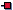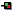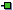Over-Loading was discussed in the section called “Over-Loading” in the context of general object-oriented programming and applies in the exact same way to the C++ language. In any C++ class the same method name may define many different operations depending on the parameters used. For example:

``` 1
2
4
5   #include<iostream>
6   #include<string>
7
8   using namespace std;
9
10   class MyMaths
11   {
12     public:
13       virtual int add(int a, int b);
14       virtual float add(float a, float b);
15       virtual string add(string a, string b);
16   };
17
18   int MyMaths::add(int a, int b)
19   {
20     return (a+b);
21   }
22
23   float MyMaths::add(float a, float b) {
24     return (a+b);
25   }
26
27   string MyMaths::add(string a, string b)
28   {
29     return (a+b);
30   }
31
32   main() {
33 	MyMaths m;
34
35 	cout << "1 + 2 = " << m.add(1,2) << endl;
36 	cout << "1.5 + 2.0 = " << m.add(1.5f,2.0f) << endl;
37 	cout << "one + two = " << m.add("one","two") << endl;
38   }
39 ```

The full source code for this example is in `overloading.cpp` In this application there are three different`add()` methods in the same class. Each method differs from the last by having different parameter argument types and so will behave slightly differently depending on the values passed. For example, the call that passes two int values will result in an int result that is the addition of the two integer values. If two string values are passed then the result is a string type result that is the concatenation of the two passed strings. So the output of this code segment will be:

```  1 + 2 = 3
1.5 + 2.0 = 3.5
one + two = onetwo
```

Note: A method cannot be distinguished by its return type solely, so the parameter list must differ in some way (types or number of arguments). Please be aware that this example is fundamentally flawed. If you look at the implementation of my`add()` methods, they all have exactly the same implementation, i.e. the statement `return (a+b)`. So, how is it possible that the "+" operator is able to work with ints, floats or even strings? Well, this is called operator overloading.

C++ allows the programmer to associate specific functionality of a class with the standard predefined operators, such as `'+','-','=','=='` etc. One class which demonstrates the use of these over-loaded operators is the`String` class, which associates the `'+'` with concatenation, `'='` to assignment etc. We can over-load the predefined operators to suit our own classes

For example, in the`Account` class we can add a `+` operator, allowing`Account` objects to be summed in an appropriate way. For example:

``` 1
2 // Operator Overloaded Account Example
3
4 #include<iostream>
5 #include<string>
6
7 using namespace std;
8
9 class Account{
10
11   protected:
12
13     int accountNumber;
14     float balance;
15     string owner;
16
17   public:
18
19     Account(string owner, float aBalance, int anAccountNumber);
20     Account(float aBalance, int anAccountNumber);
21     Account(int anAccountNumber);
22
23     Account operator + (Account);
24     bool operator == (Account);
25
26     virtual void display();
27     virtual void makeLodgement(float);
28     virtual void makeWithdrawal(float);
29 };
30
31 Account::Account(string anOwner, float aBalance, int anAccNumber):
32 	accountNumber(anAccNumber), balance(aBalance),
33 	owner (anOwner) {}
34
35 Account::Account(float aBalance, int anAccNumber) :
36 	accountNumber(anAccNumber), balance(aBalance),
37 	owner ("Not Defined") {}
38
39 Account::Account(int anAccNumber):
40 	accountNumber(anAccNumber), balance(0.0f),
41 	owner ("Not Defined") {}
42
43 Account Account::operator + (Account a)
44 {
45     return Account(owner, balance + a.balance, accountNumber);
46 }
47
48 bool Account::operator == (Account a)
49 {
50     if ((a.balance == balance) && (a.owner == owner) &&
51 		(a.accountNumber == accountNumber))
52     { return true; }
53     else return false;
54 }
55
56
57 void Account::display(){;
58     cout << "account number: " << accountNumber
59       << " has balance: " << balance << " Euro" << endl;
60     cout << "This account is owned by: " << owner << endl;
61 }
62
63 void Account::makeLodgement(float amount){
64     balance = balance + amount;
65 }
66
67 void Account::makeWithdrawal(float amount){
68     balance = balance - amount;
69 }
70
71 int main()
72 {
73   Account a = Account(10.50, 123456);
74   a.display();
75
76   Account b = Account("Derek Molloy", 35.50, 123457);
77   b.display();
78
79   Account c = Account("Derek Molloy", 35.50, 123457);
80   if (b == c) { cout << "b and c are equal!" << endl; }
81
82   Account d = b + a;
83   d.display();
84 }
85
86 ```

The full source code for this example is in `OperatorOverloadAccount.cpp`

In this example the `+` operator has been over-loaded to add the`balance` of the object on the RHS of the + to the object on the LHS. It does not add the`accountNumber` or the`owner` states (as this would not make sense). The method creates a new`Account` object and returns it from the method. This is assigned to`c`on the LHS of the assignment, when `Account d = b + a;` is executed. The output from this code is:

```
C:\My Documents\My Teaching\EE402 Notes\source_notes\cpp>

account number: 123456 has balance: 10.5 Euro
This account is owned by: Not Defined
account number: 123457 has balance: 35.5 Euro
This account is owned by: Derek Molloy
b and c are equal!
account number: 123457 has balance: 46 Euro
This account is owned by: Derek Molloy

C:\My Documents\My Teaching\EE402 Notes\source_notes\cpp>

```

In the line of code:

```	Account d = b + a;
```

We can look at the statement as two stages. In the first stage `b + a` is called. Imagine that the `+` operator was replaced by an`add()` method. You would call`b.add(a)`to add an`Account` object`a` to an`Account` object`b`. Well the `+` operator is exactly like this - you can think of it as`b.+(a)` if you like. Now in my example, rather than add the object`a` to`b`, I allowed the method to create a new object. So, the first element passed to the operator is the object you are operating on, and the second element is the parameter that you are passing.

In the second stage `d = (b + a);` the assignment sets the LHS to be equal to the new object returned from the`+` method.

The reason that the`+` method has full access to the private data of the`Account` object`a` that was passed as a parameter is because the code was written inside the`Account` class, therefore, having full private access to the states of the`Account` class.

In this example the `==` operator allows a comparison of two`Account` objects, by comparing the states of the objects to see if they are equal. It is up to the programmer to decide what defines objects that are equal. For example, in the`Account` you may decide that two`Account` objects are equal if the owner and balance are the same, or you may also require that the`accountNumber` states are also equal. The `==` operator can therefore have specific implementation in different classes.

These notes are copyright Dr. Derek Molloy, School of Electronic Engineering, Dublin City University, Ireland 2013-present. Please contact him directly before reproducing any of the content in any way.
ċ
Derek Molloy,
19 Oct 2013, 05:00
ċ
Derek Molloy,
19 Oct 2013, 05:00